# 12.1 The ellipse  (Page 9/16)

 Page 9 / 16

$4{x}^{2}+16{y}^{2}=1$

$\frac{{\left(x-2\right)}^{2}}{49}+\frac{{\left(y-4\right)}^{2}}{25}=1$

$\frac{{\left(x-2\right)}^{2}}{{7}^{2}}+\frac{{\left(y-4\right)}^{2}}{{5}^{2}}=1;\text{\hspace{0.17em}}$ Endpoints of major axis $\text{\hspace{0.17em}}\left(9,4\right),\left(-5,4\right).\text{\hspace{0.17em}}$ Endpoints of minor axis $\text{\hspace{0.17em}}\left(2,9\right),\left(2,-1\right).\text{\hspace{0.17em}}$ Foci at $\text{\hspace{0.17em}}\left(2+2\sqrt{6},4\right),\left(2-2\sqrt{6},4\right).$

$\frac{{\left(x-2\right)}^{2}}{81}+\frac{{\left(y+1\right)}^{2}}{16}=1$

$\frac{{\left(x+5\right)}^{2}}{4}+\frac{{\left(y-7\right)}^{2}}{9}=1$

$\frac{{\left(x+5\right)}^{2}}{{2}^{2}}+\frac{{\left(y-7\right)}^{2}}{{3}^{2}}=1;\text{\hspace{0.17em}}$ Endpoints of major axis $\text{\hspace{0.17em}}\left(-5,10\right),\left(-5,4\right).\text{\hspace{0.17em}}$ Endpoints of minor axis $\text{\hspace{0.17em}}\left(-3,7\right),\left(-7,7\right).\text{\hspace{0.17em}}$ Foci at $\text{\hspace{0.17em}}\left(-5,7+\sqrt{5}\right),\left(-5,7-\sqrt{5}\right).$

$\frac{{\left(x-7\right)}^{2}}{49}+\frac{{\left(y-7\right)}^{2}}{49}=1$

$4{x}^{2}-8x+9{y}^{2}-72y+112=0$

$\frac{{\left(x-1\right)}^{2}}{{3}^{2}}+\frac{{\left(y-4\right)}^{2}}{{2}^{2}}=1;\text{\hspace{0.17em}}$ Endpoints of major axis $\text{\hspace{0.17em}}\left(4,4\right),\left(-2,4\right).\text{\hspace{0.17em}}$ Endpoints of minor axis $\text{\hspace{0.17em}}\left(1,6\right),\left(1,2\right).\text{\hspace{0.17em}}$ Foci at $\text{\hspace{0.17em}}\left(1+\sqrt{5},4\right),\left(1-\sqrt{5},4\right).$

$9{x}^{2}-54x+9{y}^{2}-54y+81=0$

$4{x}^{2}-24x+36{y}^{2}-360y+864=0$

$\frac{{\left(x-3\right)}^{2}}{{\left(3\sqrt{2}\right)}^{2}}+\frac{{\left(y-5\right)}^{2}}{{\left(\sqrt{2}\right)}^{2}}=1;\text{\hspace{0.17em}}$ Endpoints of major axis $\text{\hspace{0.17em}}\left(3+3\sqrt{2},5\right),\left(3-3\sqrt{2},5\right).\text{\hspace{0.17em}}$ Endpoints of minor axis $\text{\hspace{0.17em}}\left(3,5+\sqrt{2}\right),\left(3,5-\sqrt{2}\right).\text{\hspace{0.17em}}$ Foci at $\text{\hspace{0.17em}}\left(7,5\right),\left(-1,5\right).$

$4{x}^{2}+24x+16{y}^{2}-128y+228=0$

$4{x}^{2}+40x+25{y}^{2}-100y+100=0$

$\frac{{\left(x+5\right)}^{2}}{{\left(5\right)}^{2}}+\frac{{\left(y-2\right)}^{2}}{{\left(2\right)}^{2}}=1;\text{\hspace{0.17em}}$ Endpoints of major axis $\text{\hspace{0.17em}}\left(0,2\right),\left(-10,2\right).\text{\hspace{0.17em}}$ Endpoints of minor axis $\text{\hspace{0.17em}}\left(-5,4\right),\left(-5,0\right).\text{\hspace{0.17em}}$ Foci at $\text{\hspace{0.17em}}\left(-5+\sqrt{21},2\right),\left(-5-\sqrt{21},2\right).$

${x}^{2}+2x+100{y}^{2}-1000y+2401=0$

$4{x}^{2}+24x+25{y}^{2}+200y+336=0$

$\frac{{\left(x+3\right)}^{2}}{{\left(5\right)}^{2}}+\frac{{\left(y+4\right)}^{2}}{{\left(2\right)}^{2}}=1;\text{\hspace{0.17em}}$ Endpoints of major axis $\text{\hspace{0.17em}}\left(2,-4\right),\left(-8,-4\right).\text{\hspace{0.17em}}$ Endpoints of minor axis $\text{\hspace{0.17em}}\left(-3,-2\right),\left(-3,-6\right).\text{\hspace{0.17em}}$ Foci at $\text{\hspace{0.17em}}\left(-3+\sqrt{21},-4\right),\left(-3-\sqrt{21},-4\right).$

$9{x}^{2}+72x+16{y}^{2}+16y+4=0$

For the following exercises, find the foci for the given ellipses.

$\frac{{\left(x+3\right)}^{2}}{25}+\frac{{\left(y+1\right)}^{2}}{36}=1$

Foci $\text{\hspace{0.17em}}\left(-3,-1+\sqrt{11}\right),\left(-3,-1-\sqrt{11}\right)$

$\frac{{\left(x+1\right)}^{2}}{100}+\frac{{\left(y-2\right)}^{2}}{4}=1$

${x}^{2}+{y}^{2}=1$

Focus $\text{\hspace{0.17em}}\left(0,0\right)$

${x}^{2}+4{y}^{2}+4x+8y=1$

$10{x}^{2}+{y}^{2}+200x=0$

Foci $\text{\hspace{0.17em}}\left(-10,30\right),\left(-10,-30\right)$

## Graphical

For the following exercises, graph the given ellipses, noting center, vertices, and foci.

$\frac{{x}^{2}}{25}+\frac{{y}^{2}}{36}=1$

$\frac{{x}^{2}}{16}+\frac{{y}^{2}}{9}=1$

Center $\text{\hspace{0.17em}}\left(0,0\right),\text{\hspace{0.17em}}$ Vertices $\text{\hspace{0.17em}}\left(4,0\right),\left(-4,0\right),\left(0,3\right),\left(0,-3\right),\text{\hspace{0.17em}}$ Foci $\text{\hspace{0.17em}}\left(\sqrt{7},0\right),\left(-\sqrt{7},0\right)$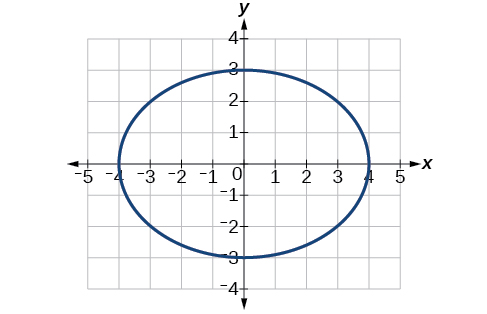$4{x}^{2}+9{y}^{2}=1$

$81{x}^{2}+49{y}^{2}=1$

Center $\text{\hspace{0.17em}}\left(0,0\right),\text{\hspace{0.17em}}$ Vertices $\text{\hspace{0.17em}}\left(\frac{1}{9},0\right),\left(-\frac{1}{9},0\right),\left(0,\frac{1}{7}\right),\left(0,-\frac{1}{7}\right),\text{\hspace{0.17em}}$ Foci $\text{\hspace{0.17em}}\left(0,\frac{4\sqrt{2}}{63}\right),\left(0,-\frac{4\sqrt{2}}{63}\right)$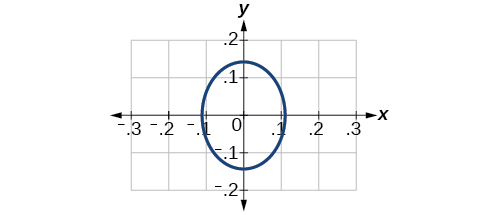$\frac{{\left(x-2\right)}^{2}}{64}+\frac{{\left(y-4\right)}^{2}}{16}=1$

$\frac{{\left(x+3\right)}^{2}}{9}+\frac{{\left(y-3\right)}^{2}}{9}=1$

Center $\text{\hspace{0.17em}}\left(-3,3\right),\text{\hspace{0.17em}}$ Vertices $\text{\hspace{0.17em}}\left(0,3\right),\left(-6,3\right),\left(-3,0\right),\left(-3,6\right),\text{\hspace{0.17em}}$ Focus $\text{\hspace{0.17em}}\left(-3,3\right)\text{\hspace{0.17em}}$

Note that this ellipse is a circle. The circle has only one focus, which coincides with the center.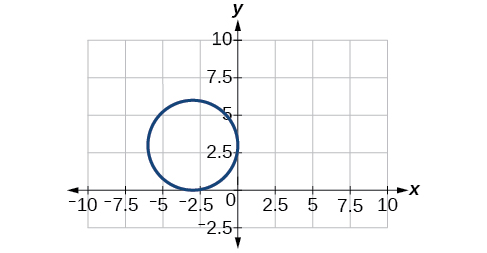$\frac{{x}^{2}}{2}+\frac{{\left(y+1\right)}^{2}}{5}=1$

$4{x}^{2}-8x+16{y}^{2}-32y-44=0$

Center $\text{\hspace{0.17em}}\left(1,1\right),\text{\hspace{0.17em}}$ Vertices $\text{\hspace{0.17em}}\left(5,1\right),\left(-3,1\right),\left(1,3\right),\left(1,-1\right),\text{\hspace{0.17em}}$ Foci $\text{\hspace{0.17em}}\left(1,1+4\sqrt{3}\right),\left(1,1-4\sqrt{3}\right)$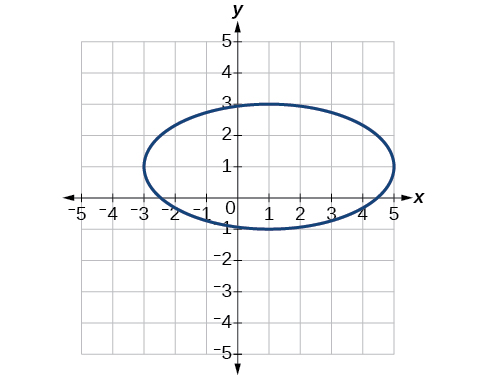${x}^{2}-8x+25{y}^{2}-100y+91=0$

${x}^{2}+8x+4{y}^{2}-40y+112=0$

Center $\text{\hspace{0.17em}}\left(-4,5\right),\text{\hspace{0.17em}}$ Vertices $\text{\hspace{0.17em}}\left(-2,5\right),\left(-6,4\right),\left(-4,6\right),\left(-4,4\right),\text{\hspace{0.17em}}$ Foci $\text{\hspace{0.17em}}\left(-4+\sqrt{3},5\right),\left(-4-\sqrt{3},5\right)$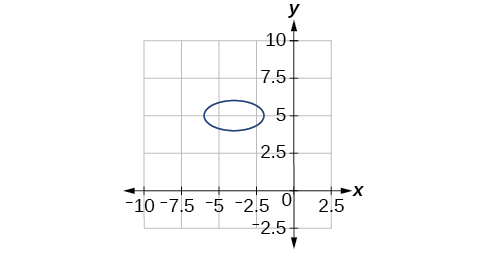$64{x}^{2}+128x+9{y}^{2}-72y-368=0$

$16{x}^{2}+64x+4{y}^{2}-8y+4=0$

Center $\text{\hspace{0.17em}}\left(-2,1\right),\text{\hspace{0.17em}}$ Vertices $\text{\hspace{0.17em}}\left(0,1\right),\left(-4,1\right),\left(-2,5\right),\left(-2,-3\right),\text{\hspace{0.17em}}$ Foci $\text{\hspace{0.17em}}\left(-2,1+2\sqrt{3}\right),\left(-2,1-2\sqrt{3}\right)$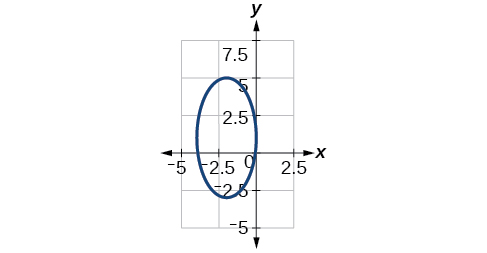$100{x}^{2}+1000x+{y}^{2}-10y+2425=0$

$4{x}^{2}+16x+4{y}^{2}+16y+16=0$

Center $\text{\hspace{0.17em}}\left(-2,-2\right),\text{\hspace{0.17em}}$ Vertices $\text{\hspace{0.17em}}\left(0,-2\right),\left(-4,-2\right),\left(-2,0\right),\left(-2,-4\right),\text{\hspace{0.17em}}$ Focus $\text{\hspace{0.17em}}\left(-2,-2\right)$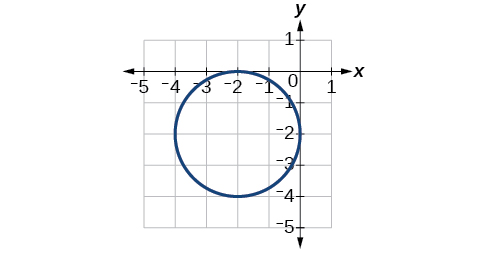For the following exercises, use the given information about the graph of each ellipse to determine its equation.

Center at the origin, symmetric with respect to the x - and y -axes, focus at $\text{\hspace{0.17em}}\left(4,0\right),$ and point on graph $\text{\hspace{0.17em}}\left(0,3\right).$

Center at the origin, symmetric with respect to the x - and y -axes, focus at $\text{\hspace{0.17em}}\left(0,-2\right),$ and point on graph $\text{\hspace{0.17em}}\left(5,0\right).$

$\frac{{x}^{2}}{25}+\frac{{y}^{2}}{29}=1$

Center at the origin, symmetric with respect to the x - and y -axes, focus at $\text{\hspace{0.17em}}\left(3,0\right),$ and major axis is twice as long as minor axis.

Center $\text{\hspace{0.17em}}\left(4,2\right)$ ; vertex $\text{\hspace{0.17em}}\left(9,2\right)$ ; one focus: $\text{\hspace{0.17em}}\left(4+2\sqrt{6},2\right)$ .

$\frac{{\left(x-4\right)}^{2}}{25}+\frac{{\left(y-2\right)}^{2}}{1}=1$

Center $\text{\hspace{0.17em}}\left(3,5\right)$ ; vertex $\text{\hspace{0.17em}}\left(3,11\right)$ ; one focus:

Center $\text{\hspace{0.17em}}\left(-3,4\right)$ ; vertex $\text{\hspace{0.17em}}\left(1,4\right)$ ; one focus: $\text{\hspace{0.17em}}\left(-3+2\sqrt{3},4\right)$

$\frac{{\left(x+3\right)}^{2}}{16}+\frac{{\left(y-4\right)}^{2}}{4}=1$

For the following exercises, given the graph of the ellipse, determine its equation.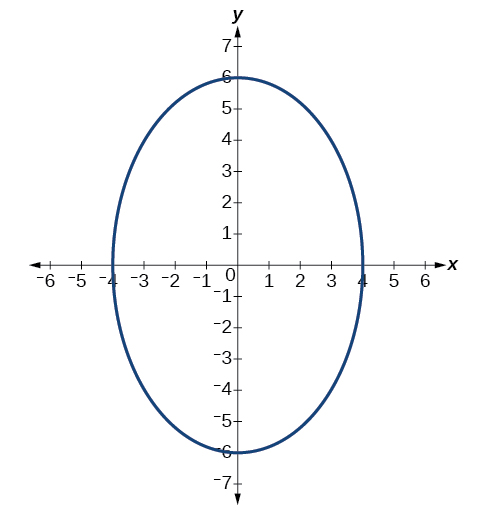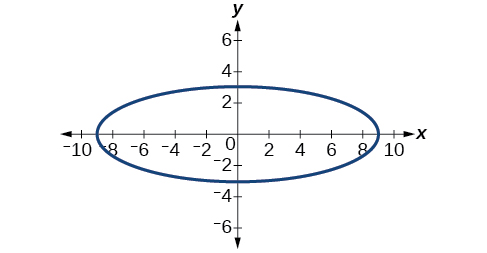$\frac{{x}^{2}}{81}+\frac{{y}^{2}}{9}=1$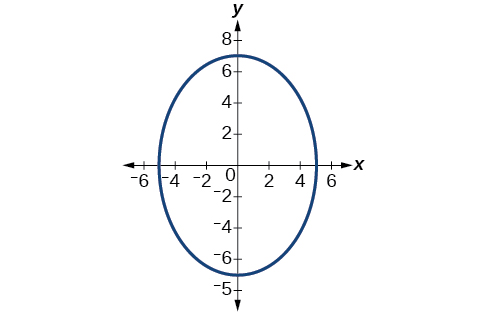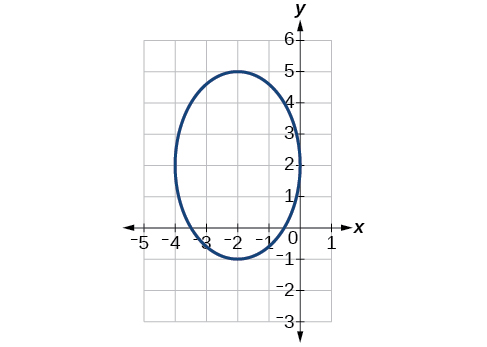$\frac{{\left(x+2\right)}^{2}}{4}+\frac{{\left(y-2\right)}^{2}}{9}=1$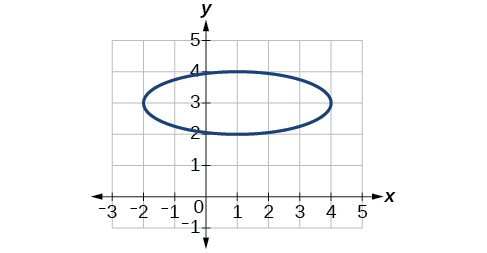## Extensions

For the following exercises, find the area of the ellipse. The area of an ellipse is given by the formula $\text{\hspace{0.17em}}\text{Area}=a\cdot b\cdot \pi .$

$\frac{{\left(x-3\right)}^{2}}{9}+\frac{{\left(y-3\right)}^{2}}{16}=1$

$\frac{{\left(x+6\right)}^{2}}{16}+\frac{{\left(y-6\right)}^{2}}{36}=1$

$\frac{{\left(x+1\right)}^{2}}{4}+\frac{{\left(y-2\right)}^{2}}{5}=1$

$4{x}^{2}-8x+9{y}^{2}-72y+112=0$

$9{x}^{2}-54x+9{y}^{2}-54y+81=0$

## Real-world applications

Find the equation of the ellipse that will just fit inside a box that is 8 units wide and 4 units high.

Find the equation of the ellipse that will just fit inside a box that is four times as wide as it is high. Express in terms of $\text{\hspace{0.17em}}h,$ the height.

$\frac{{x}^{2}}{4{h}^{2}}+\frac{{y}^{2}}{\frac{1}{4}{h}^{2}}=1$

An arch has the shape of a semi-ellipse (the top half of an ellipse). The arch has a height of 8 feet and a span of 20 feet. Find an equation for the ellipse, and use that to find the height to the nearest 0.01 foot of the arch at a distance of 4 feet from the center.

An arch has the shape of a semi-ellipse. The arch has a height of 12 feet and a span of 40 feet. Find an equation for the ellipse, and use that to find the distance from the center to a point at which the height is 6 feet. Round to the nearest hundredth.

$\frac{{x}^{2}}{400}+\frac{{y}^{2}}{144}=1$ . Distance = 17.32 feet

A bridge is to be built in the shape of a semi-elliptical arch and is to have a span of 120 feet. The height of the arch at a distance of 40 feet from the center is to be 8 feet. Find the height of the arch at its center.

A person in a whispering gallery standing at one focus of the ellipse can whisper and be heard by a person standing at the other focus because all the sound waves that reach the ceiling are reflected to the other person. If a whispering gallery has a length of 120 feet, and the foci are located 30 feet from the center, find the height of the ceiling at the center.

Approximately 51.96 feet

A person is standing 8 feet from the nearest wall in a whispering gallery. If that person is at one focus, and the other focus is 80 feet away, what is the length and height at the center of the gallery?

(x2-2x+8)-4(x2-3x+5)
sorry
Miranda
x²-2x+9-4x²+12x-20 -3x²+10x+11
Miranda
x²-2x+9-4x²+12x-20 -3x²+10x+11
Miranda
(X2-2X+8)-4(X2-3X+5)=0 ?
master
The anwser is imaginary number if you want to know The anwser of the expression you must arrange The expression and use quadratic formula To find the answer
master
The anwser is imaginary number if you want to know The anwser of the expression you must arrange The expression and use quadratic formula To find the answer
master
Y
master
master
Soo sorry (5±Root11* i)/3
master
Mukhtar
explain and give four example of hyperbolic function
What is the correct rational algebraic expression of the given "a fraction whose denominator is 10 more than the numerator y?
y/y+10
Mr
Find nth derivative of eax sin (bx + c).
Find area common to the parabola y2 = 4ax and x2 = 4ay.
Anurag
A rectangular garden is 25ft wide. if its area is 1125ft, what is the length of the garden
to find the length I divide the area by the wide wich means 1125ft/25ft=45
Miranda
thanks
Jhovie
What do you call a relation where each element in the domain is related to only one value in the range by some rules?
A banana.
Yaona
given 4cot thither +3=0and 0°<thither <180° use a sketch to determine the value of the following a)cos thither
what are you up to?
nothing up todat yet
Miranda
hi
jai
hello
jai
Miranda Drice
jai
aap konsi country se ho
jai
which language is that
Miranda
I am living in india
jai
good
Miranda
what is the formula for calculating algebraic
I think the formula for calculating algebraic is the statement of the equality of two expression stimulate by a set of addition, multiplication, soustraction, division, raising to a power and extraction of Root. U believe by having those in the equation you will be in measure to calculate it
Miranda
state and prove Cayley hamilton therom
hello
Propessor
hi
Miranda
the Cayley hamilton Theorem state if A is a square matrix and if f(x) is its characterics polynomial then f(x)=0 in another ways evey square matrix is a root of its chatacteristics polynomial.
Miranda
hi
jai
hi Miranda
jai
thanks
Propessor
welcome
jai
What is algebra
algebra is a branch of the mathematics to calculate expressions follow.
Miranda
Miranda Drice would you mind teaching me mathematics? I think you are really good at math. I'm not good at it. In fact I hate it. 😅😅😅
Jeffrey
lolll who told you I'm good at it
Miranda
something seems to wispher me to my ear that u are good at it. lol
Jeffrey
lolllll if you say so
Miranda
but seriously, Im really bad at math. And I hate it. But you see, I downloaded this app two months ago hoping to master it.
Jeffrey
which grade are you in though
Miranda
oh woww I understand
Miranda
Jeffrey
Jeffrey
Miranda
how come you finished in college and you don't like math though
Miranda
gotta practice, holmie
Steve
if you never use it you won't be able to appreciate it
Steve
I don't know why. But Im trying to like it.
Jeffrey
yes steve. you're right
Jeffrey
so you better
Miranda
what is the solution of the given equation?
which equation
Miranda
I dont know. lol
Jeffrey
Miranda
Jeffrey
answer and questions in exercise 11.2 sums
how do u calculate inequality of irrational number?
Alaba
give me an example
Chris
and I will walk you through it
Chris
cos (-z)= cos z .
cos(- z)=cos z
Mustafa
what is a algebra
(x+x)3=?
6x
Obed
what is the identity of 1-cos²5x equal to?
__john __05
Kishu
Hi
Abdel
hi
Ye
hi
Nokwanda
C'est comment
Abdel
Hi
Amanda
hello
SORIE
Hiiii
Chinni
hello
Ranjay
hi
ANSHU
hiiii
Chinni
h r u friends
Chinni
yes
Hassan
so is their any Genius in mathematics here let chat guys and get to know each other's
SORIE
I speak French
Abdel
okay no problem since we gather here and get to know each other
SORIE
hi im stupid at math and just wanna join here
Yaona
lol nahhh none of us here are stupid it's just that we have Fast, Medium, and slow learner bro but we all going to work things out together
SORIE
it's 12ByByBy RhodesBy OpenStaxBy Richley CrapoBy OpenStaxBy Jonathan LongBy Anonymous UserBy OpenStaxBy John GabrieliBy Stephen VoronBy Megan Earhart